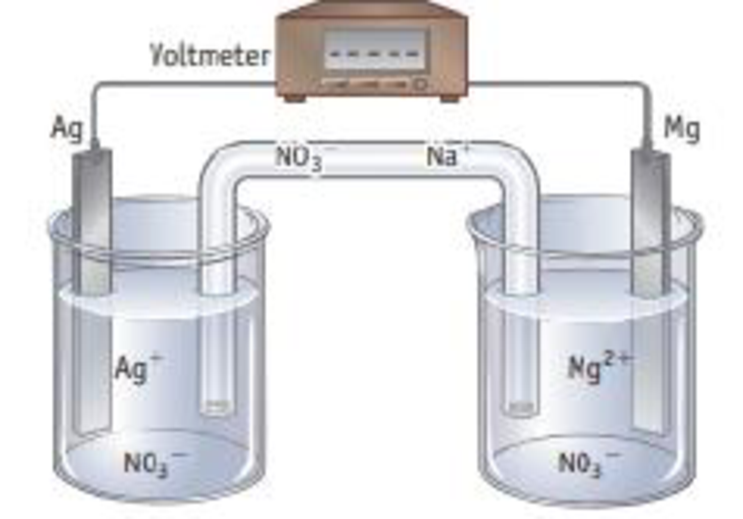Chapter 19, Problem 59GQ

Chapter
Section
Textbook Problem

Magnesium metal is oxidized, and silver ions are reduced in a voltaic cell using Mg2+(aq, 1 M) | Mg and Ag+(aq, 1 M) | Ag half-cells.(a) Label each part of the cell (b) Write equations for the half-reactions occurring at the anode and the cathode, and write an equation for the net reaction in the cell (c) Trace the movement of electrons in the external circuit. Assuming the salt bridge contains NaNO3 trace the movement of the Na+ and NO3− ions in the salt bridge that occurs when a voltaic cell produces current. Why is a salt bridge required in a cell?

(a)

Interpretation Introduction

Interpretation:

The each part of the cell has to be labelled.

Concept introduction:

Voltaic cell or Galvanic cell:

The device to produce electricity by using chemical reactions. In these divces are redox chemical reactions are occured.

A voltaic cell converts chemical energy into electrical energy.

It consists of two half cells. Each half cell consists of a metal and a solution of a salt of metal. Two half cells are connected by salt bridge.

The chemical reaction in the half cell is an oxidation reduction (redox)reactions.

For example:

Cell diagram of voltaic or galvanic cell is as follows.

Salt bridge                        Cu(s)|Cu2+(aq)  ||  Ag+(aq)|Ag(s)____________     ___________                                       Half cell             Half cell

Explanation

It consists of two half cells. Each half cell consists of a metal and a solution of a salt of metal. Two half cells are connected by salt bridge.

The chemical reaction in the ha...

(b)

Interpretation Introduction

Interpretation:

The equation for the half –reactions occurring at the anode and cathode has to be identified and write an overall reaction.

Concept introduction:

Voltaic cell or Galvanic cell:

The device to produce electricity by using chemical reactions. In these divces are redox chemical reactions are occured.

A voltaic cell converts chemical energy into electrical energy.

It consists of two half cells. Each half cell consists of a metal and a solution of a salt of metal. Two half cells are connected by salt bridge.

The chemical reaction in the half cell is an oxidation reduction (redox)reactions.

For example:

Cell diagram of voltaic or galvanic cell is as follows.

Salt bridge                        Cu(s)|Cu2+(aq)  ||  Ag+(aq)|Ag(s)____________     ___________                                       Half cell             Half cell

(c)

Interpretation Introduction

Interpretation:

The requirement of salt bridge in the cell has to be explained.

Concept introduction:

Voltaic cell or Galvanic cell:

The device to produce electricity by using chemical reactions. In these divces are redox chemical reactions are occured.

A voltaic cell converts chemical energy into electrical energy.

It consists of two half cells. Each half cell consists of a metal and a solution of a salt of metal. Two half cells are connected by salt bridge.

The chemical reaction in the half cell is an oxidation reduction (redox)reactions.

For example:

Cell diagram of voltaic or galvanic cell is as follows.

Salt bridge                        Cu(s)|Cu2+(aq)  ||  Ag+(aq)|Ag(s)____________     ___________                                       Half cell             Half cell

Still sussing out bartleby?

Check out a sample textbook solution.

See a sample solution

The Solution to Your Study Problems

Bartleby provides explanations to thousands of textbook problems written by our experts, many with advanced degrees!

Get Started